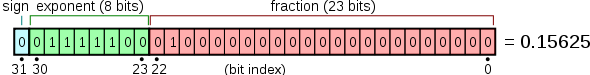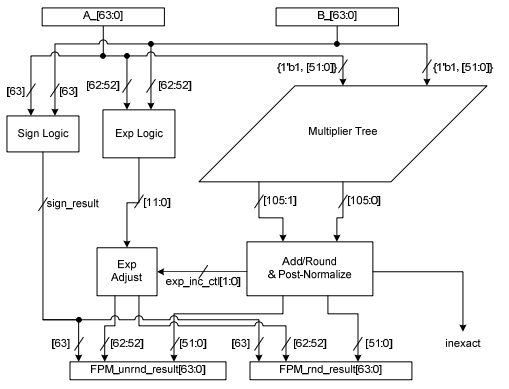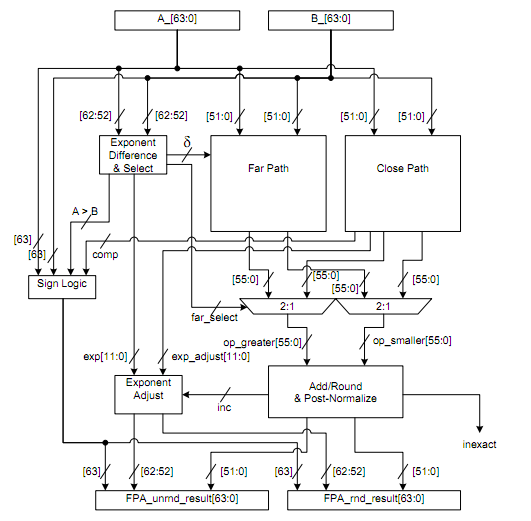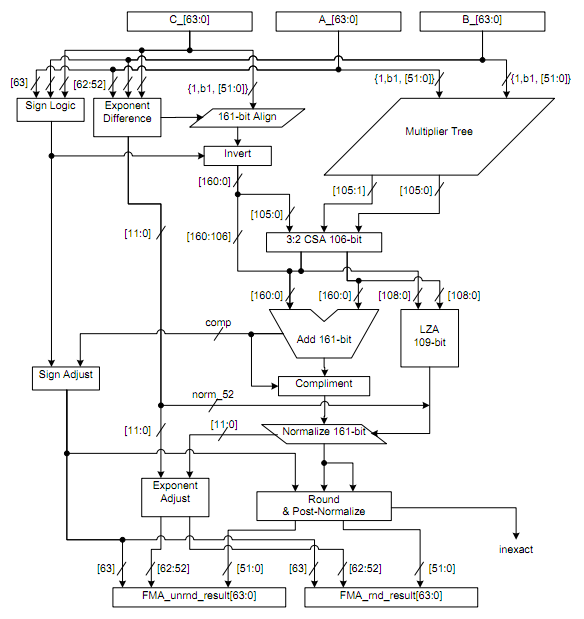by Jasper Bekkers

### Categories

• posts

To me, programming has always been an exercise in understanding blackboxes. About taking systems apart and figuring out their internal workings. And although this teaches you about how other programmers think and work it does take away some of the amazement you have when seeing a cleverly written piece of code.

For me, and for a lot of other programmers, floating point numbers have for the longest time been one of these blackboxes. There is a lot of (good and bad) information on the web about floating points, most of it describes the data format, how the bits are interpreted, what epsilon values you should use or how to deal with accuracy issues in floats. Hardly any article talks about where all of this actually comes from or how fundamental floating point operations are implemented.

So in this article I will talk about how some of these operations are implemented, specifically multiplication, addition and fused-multiply-add. I won’t talk about decimal-to-float conversions, float-to-double or float-to-int casts, division, comparisons or trigonometry functions. If you’re interested in these I suggest taking a look at John Hauser’s excellent SoftFloat library listed below. It’s the same library I’ve used to borrow the code samples in this article from.

For convenience sake I’ll also show an image of the floating point data layout taken from wikipedia because this might help explain some of the magic numbers and masks used in the code below. The hardware diagrams are taken from the “Floating-Point Fused Multiply-Add Architectures” paper linked below and are diagrams for double precision implementations (this due to me being unable to produce these pretty pictures myself). Keep that in mind when reading them.# Multiplication

The way IEEE 754 multiplication works is identical to how it works for regular scientific notation. Simply multiply the coefficients and add the exponents. However, because this is done in hardware we have some extra constraints, such as overflow and rounding, to take into account. These extra constraints are what make floats appear so ‘fuzzy’ to some.

1. Check if either of the operands (A and B) are zero (early out)
2. Check for potential exponent overflow and throw corresponding overflow errors
3. Compute sign as Csign = Asign XOR Bsign
4. Compute the exponent Cexponent = Aexponent + Bexponent - 127
5. Compute mantissa Cmantissa = Amantissa * Bmantissa (23-bit integer multiply) and round the result according to the currently set rounding mode.
6. If Cmantissa has overflown, normalize results (Cmantissa <<= 1, Cexponent -= 1)
f32 float32_mul(f32 a, f32 b)
{
// extract mantissa, exponent and sign
u32 aFrac = a & 0x007FFFFF;
u32 bFrac = b & 0x007FFFFF;

u32 aExp = (a >> 23) & 0xFF;
u32 bExp = (b >> 23) & 0xFF;

u32 aSign = a >> 31;
u32 bSign = b >> 31;

// compute sign bit
u32 zSign = aSign ^ bSign;

// removed: handle edge conditions where the exponent is about to overflow

// compute exponent
u32 zExp = aExp + bExp - 0x7F;

// add implicit 1' bit
aFrac = (aFrac | 0x00800000) << 7;
bFrac = (bFrac | 0x00800000) << 8;

u64 zFrac = (u64)aFrac * (u64)bFrac;

u32 zFrac0 = zFrac >> 32;
u32 zFrac1 = zFrac & 0xFFFFFFFF;

// check if we overflowed into more than 23-bits and handle accordingly
zFrac0 |= (zFrac1 != 0);
if(0 <= (i32)(zFrac0 << 1))
{
zFrac0 <<= 1;
zExp--;
}

// reconstruct the float; I've removed the rounding code and just truncate
return (zSign << 31) | ((zExp << 23) + (zFrac >> 7));
}Again, the steps for floating point addition are based on calculating with scientific notation. First you align the exponents, then you add the mantissas. The alignment step is the reason for the big inaccuracies with adding small and large numbers together.

1. Align binary point
1. If Aexponent > Bexponent Then do mantissa >>= 1 until Bmantissa * 2^(Bexponent - Aexponent)
2. If Bexponent > Aexponent Then do Amantissa >>= 1 until Amantissa * 2^(Aexponent - Bexponent)
3. Compute sum of aligned mantissas
1. Amantissa * 2^(Aexponent - Bexponent) + Bmantissa
2. Or Bmantissa * 2^(Bexponent - Aexponent) + Amantissa
3. Normalized and round results
4. Check for exponent overflow and throw corresponding overflow errors
5. If Cmantissa is zero set the entire float to zero to return a ‘correct’ 0 float.
// implementation only works with a and b of equal sign
// if a and b are of different sign, we call float32_sub instead
// look at the SoftFloat source-code for specifics.
static f32 float32_add(f32 a, f32 b)
{
int zExp;
u32 zFrac;

u32 aFrac = a & 0x007FFFFF;
u32 bFrac = b & 0x007FFFFF;

int aExp = (a >> 23) & 0xFF;
int bExp = (b >> 23) & 0xFF;

u32 aSign = a >> 31;
u32 bSign = b >> 31;

u32 zSign = aSign;

int expDiff = aExp - bExp;
aFrac <<= 6;
bFrac <<= 6;

// align exponents if needed
if(expDiff > 0)
{
if(bExp == 0) --expDiff;
else bFrac |= 0x20000000;

bFrac = shift32RightJamming(bFrac, expDiff);
zExp = aExp;
}
else if(expDiff < 0)
{
if(aExp == 0) ++expDiff;
else aFrac |= 0x20000000;

aFrac = shift32RightJamming(aFrac, -expDiff);
zExp = bExp;
}
else if(expDiff == 0)
{
if(aExp == 0) return (zSign << 31) | ((aFrac + bFrac) >> 13);

zFrac = 0x40000000 + aFrac + bFrac;
zExp = aExp;

return (zSign << 31) | ((zExp << 23) + (zFrac >> 7));
}

aFrac |= 0x20000000;
zFrac = (aFrac + bFrac) << 1;
--zExp;

if((i32)zFrac < 0)
{
zFrac = aFrac + bFrac;
++zExp;
}

// reconstruct the float; I've removed the rounding code and just truncate
return (zSign << 31) | ((zExp << 23) + (zFrac >> 7));
}

// for reference
static u32 shift32RightJamming(int a, int count)
{
if(count == 0) return a;
else if(count < 32) return (a >> count) | ((a << ((-count) & 31)) != 0);
else return a != 0;
}
`

An overview of floating point addition hardware. The implementation will make a distinction between adding numbers where the exponent differs (the far path) and numbers where the exponent is the same (the close path), much like the implementation above.The multiply-add operation is basically a combination of both of these operations that is as efficient or more efficient to implement in hardware as both operations separately. The primary difference in operation is (as long as it’s not a pseudo-fma) is the fact that there is only one rounding operation done at the end of the result, instead of one in the multiply and the add circuits (steps 3 and 4 respectively).

Some, if not most, SIMD architectures on current-gen platforms are actually built around just the fused-multiply-add and don’t have regular multiply or addition hardware (they’ll just insert identity constants into one of the three operands) a simple give-away for this is usually that the cycle count for these operations is identical in each case.